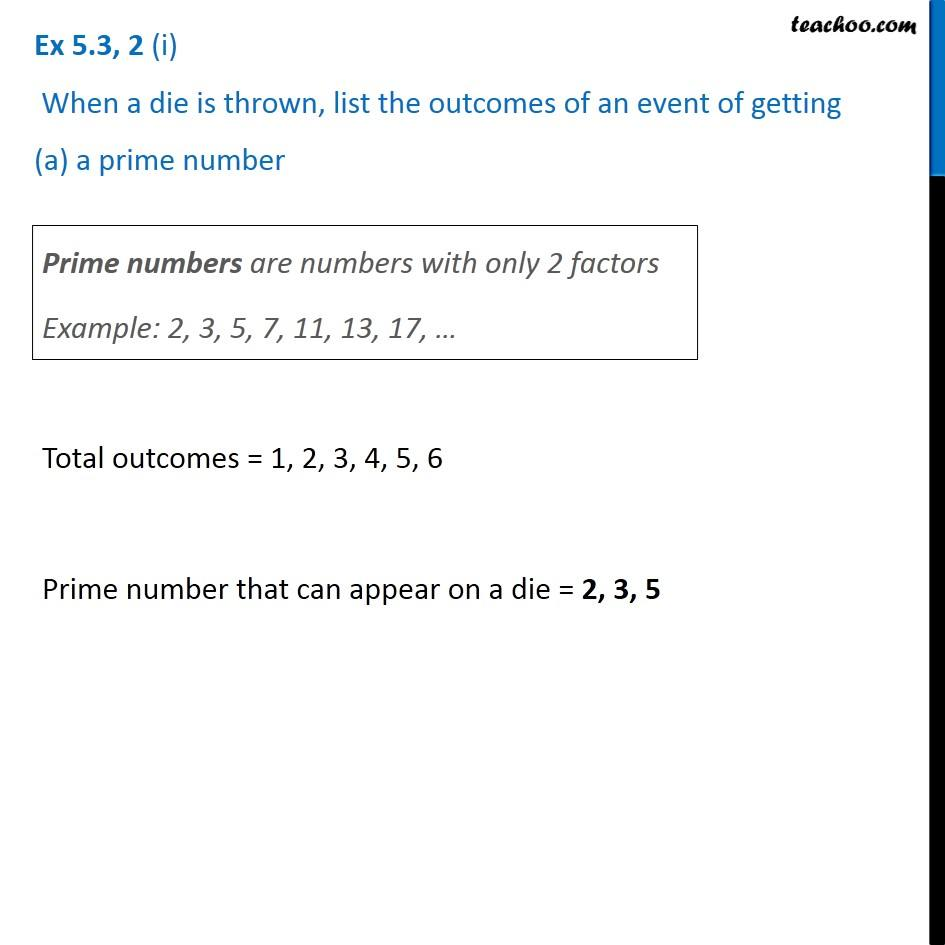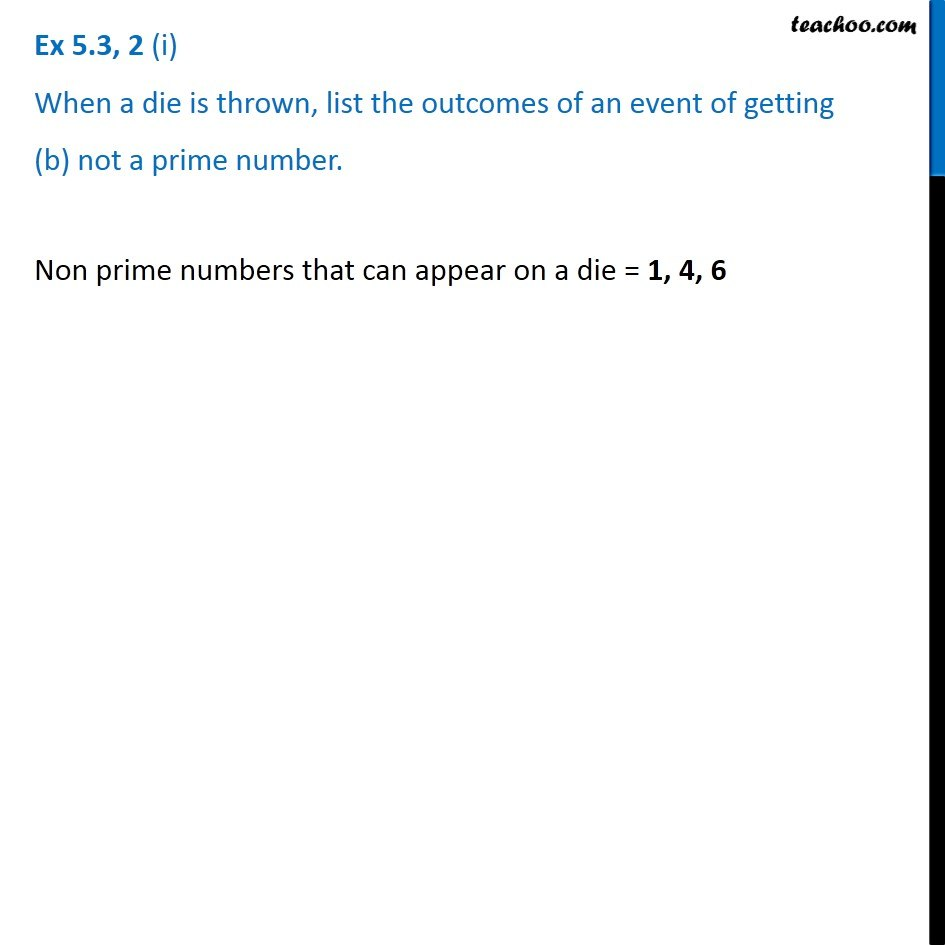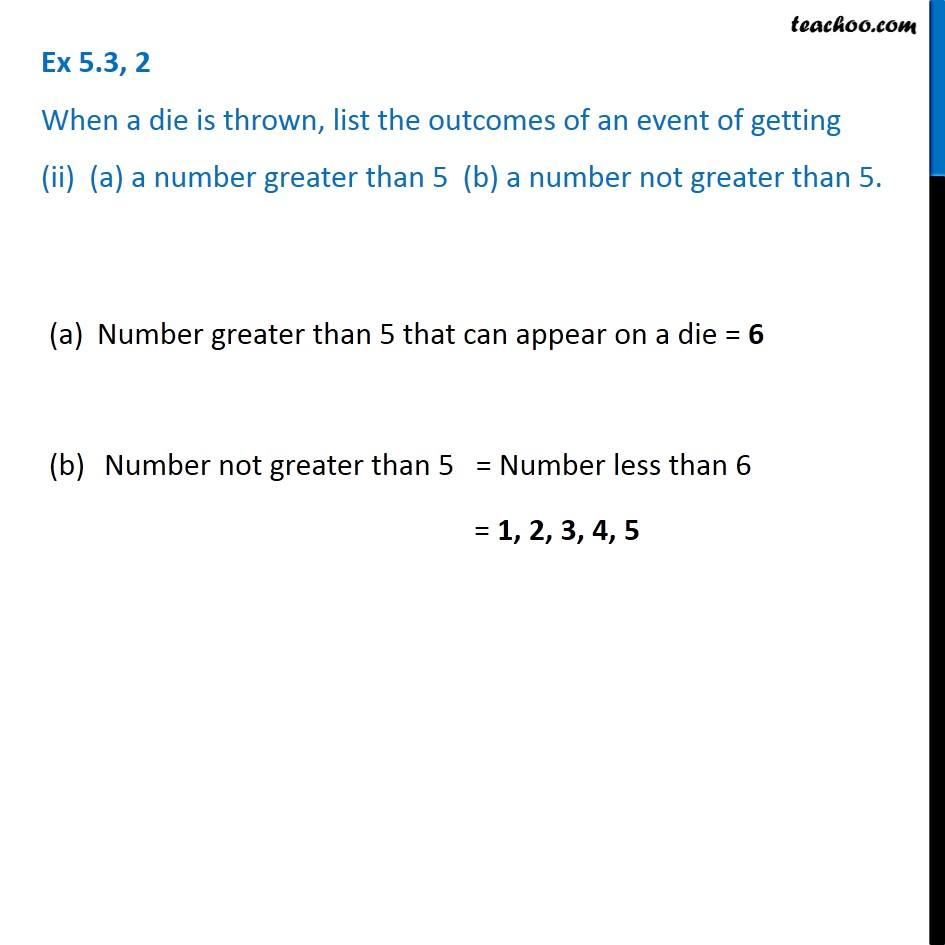Probability

Chapter 5 Class 8 Data Handling
Concept wiseIntroducing your new favourite teacher - Teachoo Black, at only ₹83 per month

### Transcript

Ex 5.3, 2 (i) When a die is thrown, list the outcomes of an event of getting (a) a prime number Prime numbers are numbers with only 2 factors Example: 2, 3, 5, 7, 11, 13, 17, … Total outcomes = 1, 2, 3, 4, 5, 6 Prime number that can appear on a die = 2, 3, 5 Ex 5.3, 2 (i) When a die is thrown, list the outcomes of an event of getting (b) not a prime number. Non prime numbers that can appear on a die = 1, 4, 6 Ex 5.3, 2 When a die is thrown, list the outcomes of an event of getting (ii) (a) a number greater than 5 (b) a number not greater than 5. Number greater than 5 that can appear on a die = 6 Number not greater than 5 = Number less than 6 = 1, 2, 3, 4, 5